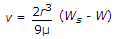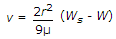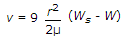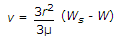# Civil Engineering - Hydraulics

Exercise : Hydraulics - Section 2
21.
Flow in pipes is laminar if Reynold number is
less than 2100
more than 3000
between 2100 and 3000
none of these.
Explanation:
No answer description is available. Let's discuss.

22.
The terminal velocity (v) of a sphere of radius r and specific weight W, which travels vertically downwards in a liquid of viscosity μ and specific weight W, isExplanation:
No answer description is available. Let's discuss.

23.
In flow, the liquid particles may possess
potential energy
kinetic energy
pressure energy
all the above.
Explanation:
No answer description is available. Let's discuss.

24.
Euler's equation for the motion of liquids assumes that
fluid is viscous
fluid is homogeneous and incompressible
velocity of flow is non-uniform over the section
flow is unsteady along the stream line.